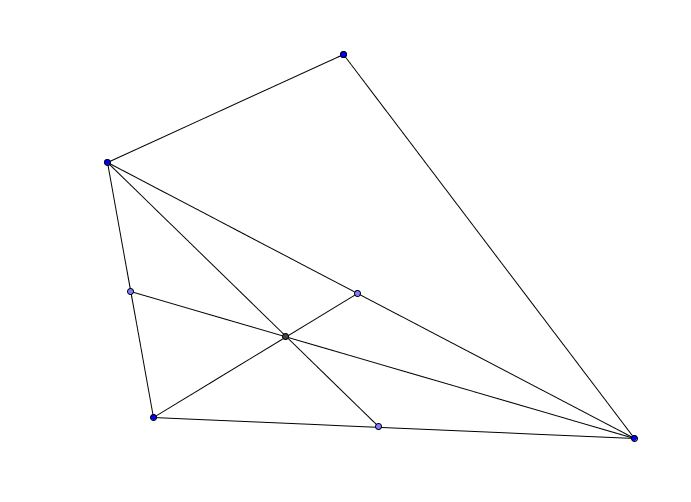Tangle with triangle

Geometry Level 5Let $\Delta ABC$ be a triangle with vertices $A,~B~\& ~C$ and $D,~E~\&~F$ be the midpoint of sides $AB,~BC~\&~CA$ respectively.

If $\color{#69047E}{AE~=~2.5}$, $\color{#EC7300}{CD~=~\sqrt{13}}$ and $\color{#20A900}{BF~=~\dfrac{\sqrt{73}}{2}}$, and $P$ be the image(reflection) of point $A$ about side $BC$.

Then find the area of the quadrilateral $\color{#D61F06}{ABPC}$

Note the given picture is not necessarily correct.

×